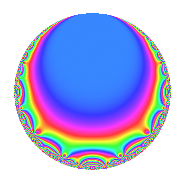# Properties

 Label 4005.2.a.gLevel 4005 Weight 2 Character orbit 4005.a Self dual Yes Analytic conductor 31.980 Analytic rank 0 Dimension 2 CM No Inner twists 1

# Related objects

## Newspace parameters

 Level: $$N$$ = $$4005 = 3^{2} \cdot 5 \cdot 89$$ Weight: $$k$$ = $$2$$ Character orbit: $$[\chi]$$ = 4005.a (trivial)

## Newform invariants

 Self dual: Yes Analytic conductor: $$31.9800860095$$ Analytic rank: $$0$$ Dimension: $$2$$ Coefficient field: $$\Q(\sqrt{17})$$ Coefficient ring: $$\Z[a_1, a_2]$$ Coefficient ring index: $$1$$ Fricke sign: $$-1$$ Sato-Tate group: $\mathrm{SU}(2)$

## $q$-expansion

Coefficients of the $$q$$-expansion are expressed in terms of $$\beta = \frac{1}{2}(1 + \sqrt{17})$$. We also show the integral $$q$$-expansion of the trace form.

 $$f(q)$$ $$=$$ $$q + \beta q^{2} + ( 2 + \beta ) q^{4} - q^{5} + ( 4 + \beta ) q^{8} +O(q^{10})$$ $$q + \beta q^{2} + ( 2 + \beta ) q^{4} - q^{5} + ( 4 + \beta ) q^{8} -\beta q^{10} -4 q^{11} + 2 q^{13} + 3 \beta q^{16} + ( -4 + 2 \beta ) q^{17} + ( -2 + 2 \beta ) q^{19} + ( -2 - \beta ) q^{20} -4 \beta q^{22} + ( 3 + \beta ) q^{23} + q^{25} + 2 \beta q^{26} + ( -5 + 3 \beta ) q^{29} + ( 2 + 2 \beta ) q^{31} + ( 4 + \beta ) q^{32} + ( 8 - 2 \beta ) q^{34} + 10 q^{37} + 8 q^{38} + ( -4 - \beta ) q^{40} + ( 5 - 3 \beta ) q^{41} + 4 \beta q^{43} + ( -8 - 4 \beta ) q^{44} + ( 4 + 4 \beta ) q^{46} + 8 q^{47} -7 q^{49} + \beta q^{50} + ( 4 + 2 \beta ) q^{52} + ( -2 + 4 \beta ) q^{53} + 4 q^{55} + ( 12 - 2 \beta ) q^{58} + ( -5 + \beta ) q^{59} + ( 8 - 2 \beta ) q^{61} + ( 8 + 4 \beta ) q^{62} + ( 4 - \beta ) q^{64} -2 q^{65} + ( -3 - 5 \beta ) q^{67} + 2 \beta q^{68} + ( 4 - 4 \beta ) q^{71} + ( -5 + 3 \beta ) q^{73} + 10 \beta q^{74} + ( 4 + 4 \beta ) q^{76} + ( -5 + \beta ) q^{79} -3 \beta q^{80} + ( -12 + 2 \beta ) q^{82} + ( 3 - 3 \beta ) q^{83} + ( 4 - 2 \beta ) q^{85} + ( 16 + 4 \beta ) q^{86} + ( -16 - 4 \beta ) q^{88} - q^{89} + ( 10 + 6 \beta ) q^{92} + 8 \beta q^{94} + ( 2 - 2 \beta ) q^{95} + ( -11 + \beta ) q^{97} -7 \beta q^{98} +O(q^{100})$$ $$\operatorname{Tr}(f)(q)$$ $$=$$ $$2q + q^{2} + 5q^{4} - 2q^{5} + 9q^{8} + O(q^{10})$$ $$2q + q^{2} + 5q^{4} - 2q^{5} + 9q^{8} - q^{10} - 8q^{11} + 4q^{13} + 3q^{16} - 6q^{17} - 2q^{19} - 5q^{20} - 4q^{22} + 7q^{23} + 2q^{25} + 2q^{26} - 7q^{29} + 6q^{31} + 9q^{32} + 14q^{34} + 20q^{37} + 16q^{38} - 9q^{40} + 7q^{41} + 4q^{43} - 20q^{44} + 12q^{46} + 16q^{47} - 14q^{49} + q^{50} + 10q^{52} + 8q^{55} + 22q^{58} - 9q^{59} + 14q^{61} + 20q^{62} + 7q^{64} - 4q^{65} - 11q^{67} + 2q^{68} + 4q^{71} - 7q^{73} + 10q^{74} + 12q^{76} - 9q^{79} - 3q^{80} - 22q^{82} + 3q^{83} + 6q^{85} + 36q^{86} - 36q^{88} - 2q^{89} + 26q^{92} + 8q^{94} + 2q^{95} - 21q^{97} - 7q^{98} + O(q^{100})$$

## Embeddings

For each embedding $$\iota_m$$ of the coefficient field, the values $$\iota_m(a_n)$$ are shown below.

For more information on an embedded modular form you can click on its label.

Label $$\iota_m(\nu)$$ $$a_{2}$$ $$a_{3}$$ $$a_{4}$$ $$a_{5}$$ $$a_{6}$$ $$a_{7}$$ $$a_{8}$$ $$a_{9}$$ $$a_{10}$$
1.1
 −1.56155 2.56155
−1.56155 0 0.438447 −1.00000 0 0 2.43845 0 1.56155
1.2 2.56155 0 4.56155 −1.00000 0 0 6.56155 0 −2.56155
 $$n$$: e.g. 2-40 or 990-1000 Significant digits: Format: Complex embeddings Normalized embeddings Satake parameters Satake angles

## Inner twists

This newform does not admit any (nontrivial) inner twists.

## Atkin-Lehner signs

$$p$$ Sign
$$3$$ $$-1$$
$$5$$ $$1$$
$$89$$ $$1$$

## Hecke kernels

This newform can be constructed as the intersection of the kernels of the following linear operators acting on $$S_{2}^{\mathrm{new}}(\Gamma_0(4005))$$:

 $$T_{2}^{2} - T_{2} - 4$$ $$T_{7}$$ $$T_{11} + 4$$